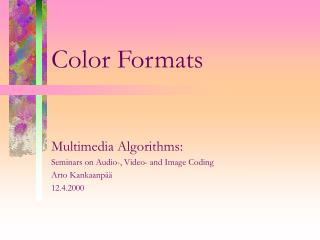Download PresentationColor Formats

# Color Formats

Download Presentation## Color Formats

- - - - - - - - - - - - - - - - - - - - - - - - - - - E N D - - - - - - - - - - - - - - - - - - - - - - - - - - -
##### Presentation Transcript

1. Color Formats Multimedia Algorithms: Seminars on Audio-, Video- and Image Coding Arto Kankaanpää 12.4.2000

2. Color Space • Means of uniquely specifying a color • Coordinate system (Color Coordinate), where each unique coordinate specifies an unique color

3. RGB Color Space • Based on Red, Green and Blue components • Basic choise for computer graphics • Not very efficient use of bandwidth • Needs Gamma-correction for CRTs

4. RGB Color Space (cont.) Gamma Correction • Compensates nonlinearity of CRTs • Correction is usually made in signal input devices (eg. cameras) to minimize the cost of output devices (eg. monitors and TVs) • Typical value for PAL/SECAM is 2.8 and 2.2 for NTSC Display characteristics Gamma correction

5. Based on Black-and-White component ( Y ) and color information ( U and V ) Used in NTSC, PAL and SECAM composite color TV standards Derived from gamma-corrected RGB signal U and V subsampled to reduse bitrate YUV Color Space { Y = 0.299R’ + 0.587G’ + 0.114B’ U =-0.147R’ - 0.289G’ + 0.436B’ V = 0.615R’ - 0.515G’ - 0.100B’ { Y = 0.299R’ + 0.587G’ + 0.114B’ U = 0.492 ( B’ - Y ) V = 0.877 ( R’ - Y )

6. YIQ Color Space • I stands for in-phase • Q stands for quadrature-phase • Derived from YUV • Optional in NTSC standard { Y = 0.299R’+0.587G’+0.114B’ I = 0.596R’-0.275G’-0.321B’ = 0.736( R’-Y ) -0.268( B’-Y ) Q = 0.212R’-0.523G’+0.211B’ = 0.478( R’-Y )+0.413( B’-Y ) • I and Q can also be obtained by advancing the phase of U and V signals [ ] [ ] [ ] [ ] I Q 0 1 1 0 cos( 33 ) sin( 33 ) -sin( 33 ) cos( 33 ) U V =

7. Was developed as part of ITU-R BT.601 digital video component standard YCbCr signals are scaled and offsetted from YUV Used by most image compression standards (JPEG, H.261, MPEG) Several sampling formats such as: 4:4:4, 4:2:2 and 4:1:1 (also 4:2:0) Sampling format implies the proportions of sampling rates of Y, Cb and Cr YCbCr Color Space

8. YCbCr Color Space (cont.) • YCbCr signals are obtained from gamma-corrected RGB { Y = 0.299R’ + 0.587G’ + 0.114B’ Cb = -0.169R’ - 0.331G’ + 0.500B’ Cr = 0.500R’ - 0.419G’ - 0.081B’ • The color difference signals are given by: • The values for (B-Y) have a range of ±0.886 and for (R-Y) a range of ±0.701; the values for Y have a range of 0 to 1 { (B - Y) = -0.299R’ - 0.587G’ + 0.886B’ (R - Y) = 0.701R’ - 0.587G’ - 0.114B’

9. YCbCr Color Space (cont.2) • Given that luminance Y is to occupy 220 levels (16 to 235) and color-difference 224 levels (16 to 240, zero being value 128). They have to be scaled to get the decimal values • Corresponding level number after quantization is rounded to the nearest integer • Having integer values is useful for most of the applications, where 8-bit binary encoding is adopted { Y’ = 219 Y + 16 Cb’ = 224 [0.564(B-Y)]+128 = 126(B-Y)+128 Cr’ = 224 [0.713(R-Y)]+128 = 160(R-Y)+128

10. SMPTE 240M Color Space • Developed to standardize the production of 1125/60 high-definition source material (mainly HDTV) in the US • The color components ( Y, Pb , Pr ) are derived from gamma-corrected RGB signals { Y = 0.212 R’ + 0.701 G’ + 0.087 B’ Pb= -0.115 R’ - 0.384 G’+0.500 B’ = (B’-Y)/1.826 Pr = 0.500 R’ - 0.445 G’ - 0.055 B’ = (R’-Y)/1.576

11. CMYeK Color Space • Cyan, Magneta, Yellow and blacK • Subtractive color model • Black is used for gray components to save ink on printers • Ideally CMYeK is complement of RGB [ ] [ ] [ ] CMYe 111 RGB _ =

12. Hue, Saturation and Value/Lightness/Intensity Developed to be more intuitive in manipulating colors Designed to approximate the way humans perceive and interpret color HSL is similar to HSI; term Lightness is used instead of Intensity Difference between HSI and HSV is in the computation of brightness component (I or V) HSI is best for traditional image processing (convolution, equalization, histograms, ... ) HSI, HSL and HSV Color Space

13. CIE 1976 (L*a*b*) Color Space • Based on CIE 1931 • Device independent • Linear three dimensional space • L* is lightness (Z-axis) • a* is redness-greeness • b* is yellowness-blueness { L* = 116 (Y/Yn)1/3 - 16 a* = 500 [ (X/Xn)1/3 - (Y/Yn)1/3 ] b* = 200 [ (Y/Yn)1/3 - (Z/Zn)1/3 ] CIE Chromaticity Chart

14. CIE 1976 (L*a*b*) (cont.) • All other color spaces are subspaces of CIE 1931 • A subset of CIE space is usually called gamut • CIELAB was developed to be perceptualy linear, which is valuable property for machine vision • CIELAB is also used by image processing applications (eg. Adobe Photoshop)